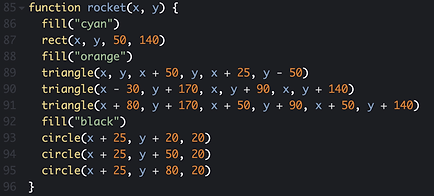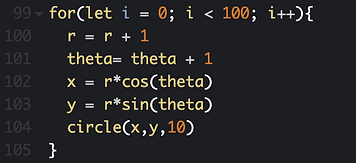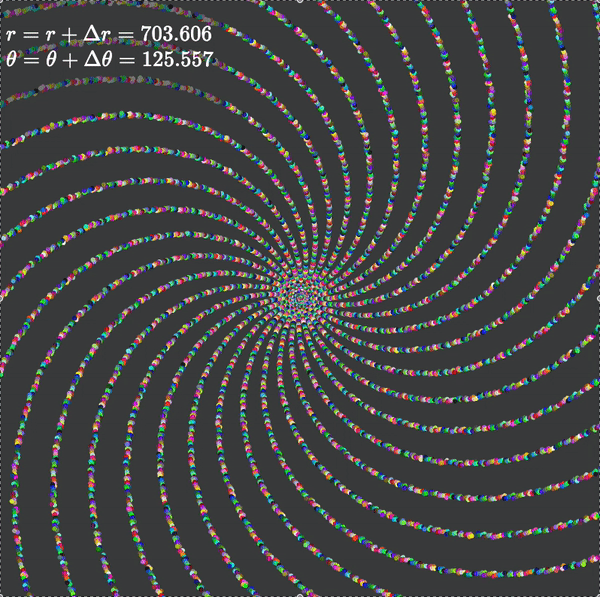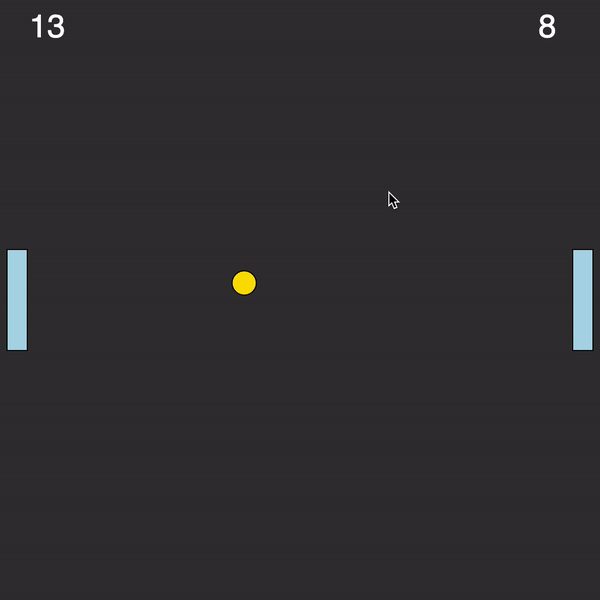# Strive Hackathon

Below is the curriculum for the first few Strive classes and Strive bootcamp. Each project becomes progressively harder and teaches more complex coding and math concepts. Depending on the student's age, prior coding experience and mathematical abilities, students will proceed through these classes at different rates. A middle school student can expect to finish the first 2 or 3 projects in the first 5 classes. A high school student with prior coding knowledge can expect to finish all 5 projects in 5 classes.

## Rocket## Day 1 | Project 1

In this project, students build a rocket that can be controlled with the arrow keys on their keyboard. They can also change the background colour using sliders controlling the red, green and blue values of the keyboard. This is a phenomenal introduction project to coding, it covers topics like variables and user input. The math topics include co-ordinate geometry, algebra and properties of shapes.## Day 2 | Project 2In this project, students create a spiral visualisation. This project is an example of a new category of mathematical art called generative art. Students learn about transformations, rotations and translations which are the building blocks of linear algebra. We also cover the properties of spirals and circles and show how they appear everywhere in nature from solar systems to shells and flowers.

## Day 3 | Project 3In this project, students learn about one of math's most fundamental formula: The Pythagoras theorem, students learn what this formula means, how it is used in computers, and it's applications in the real world. For example, Google Maps uses the Pythagoras Theorem to calculate the distance from your home to school. In the process, students learn about math topics like the distance formula, the midpoint formula, squares and square roots.

## Day 4 | Project 4A cardioid (from the Greek καρδία "heart") is a plane curve traced by a point on the perimeter of a circle that is rolling around a fixed circle of the same radius. It can also be defined as an epicycloid having a single cusp. It is also a type of  and an inverse curve of the parabola with the focus as the center of inversion.## Day 5 | Project 5Students recreate a two-player version of the game pong. Despite looking simple the project can be quite challenging. Students need to be able to model a ball and move the paddles via keyboard input. In order to do so, students need to understand vectors, trigonometry and speed.### Register Now

##### \$550
• 7.5 hours of 1-1 lessons.

• Dedicated Strive profile that can be used for job and university applications.